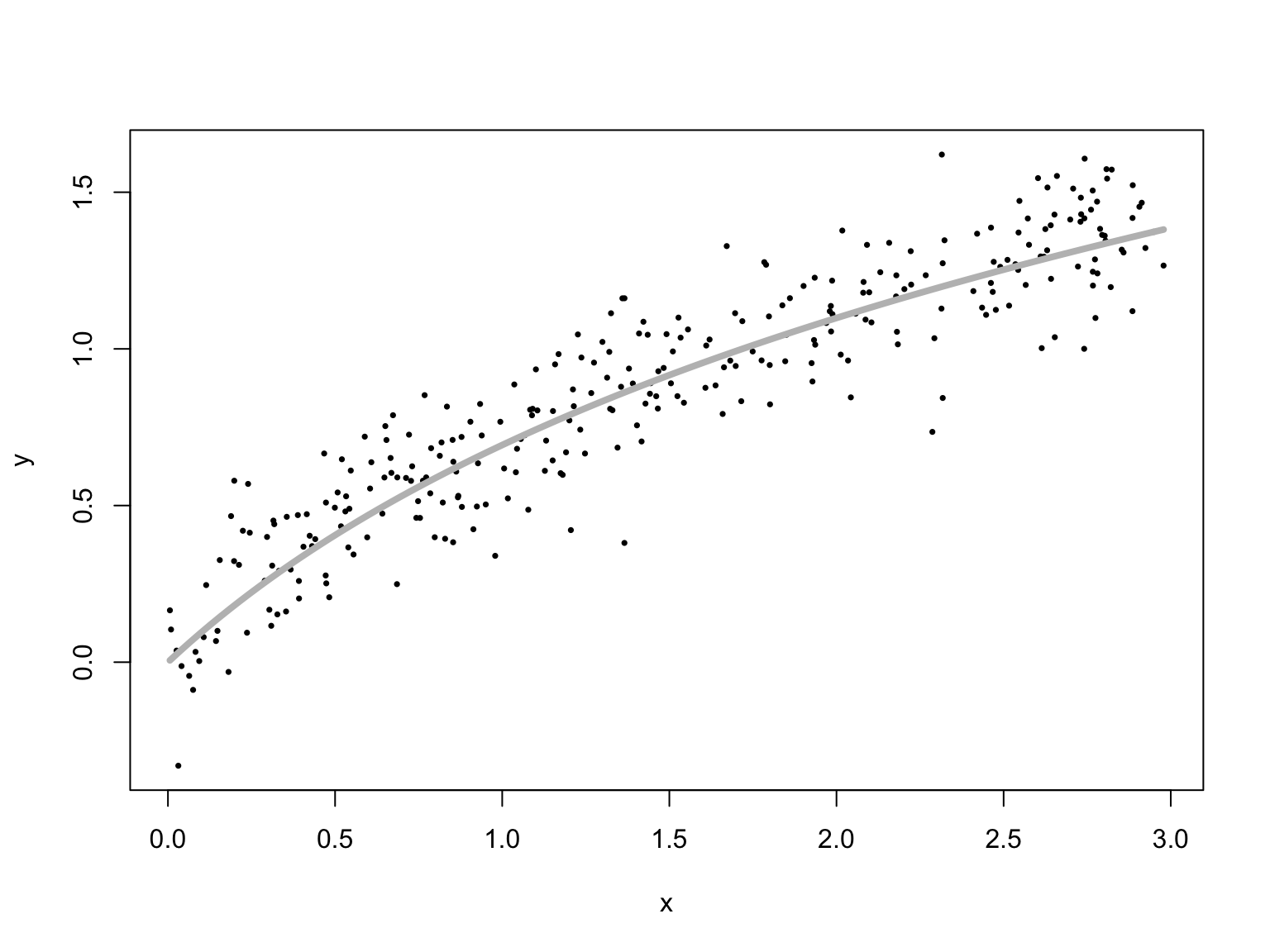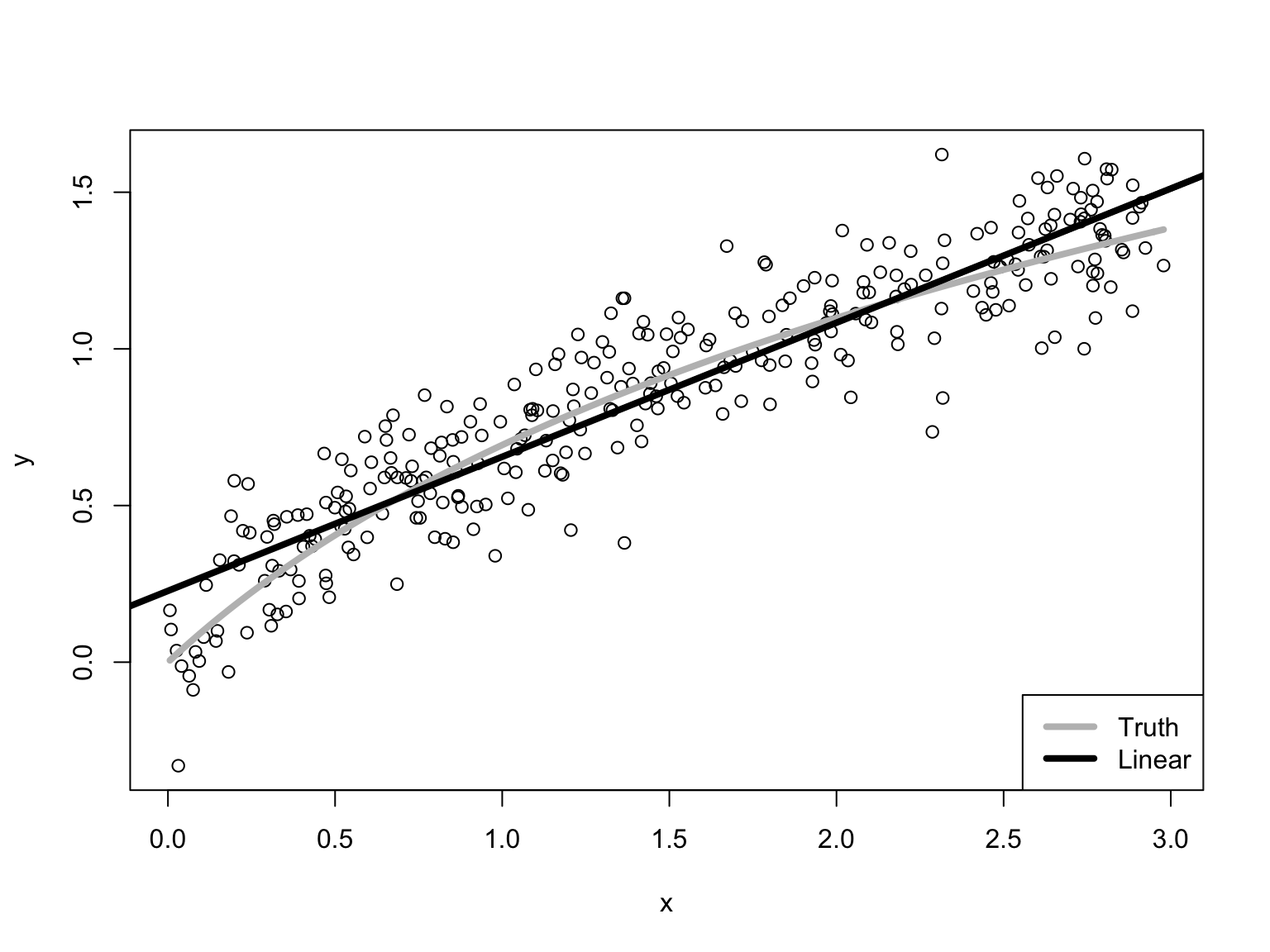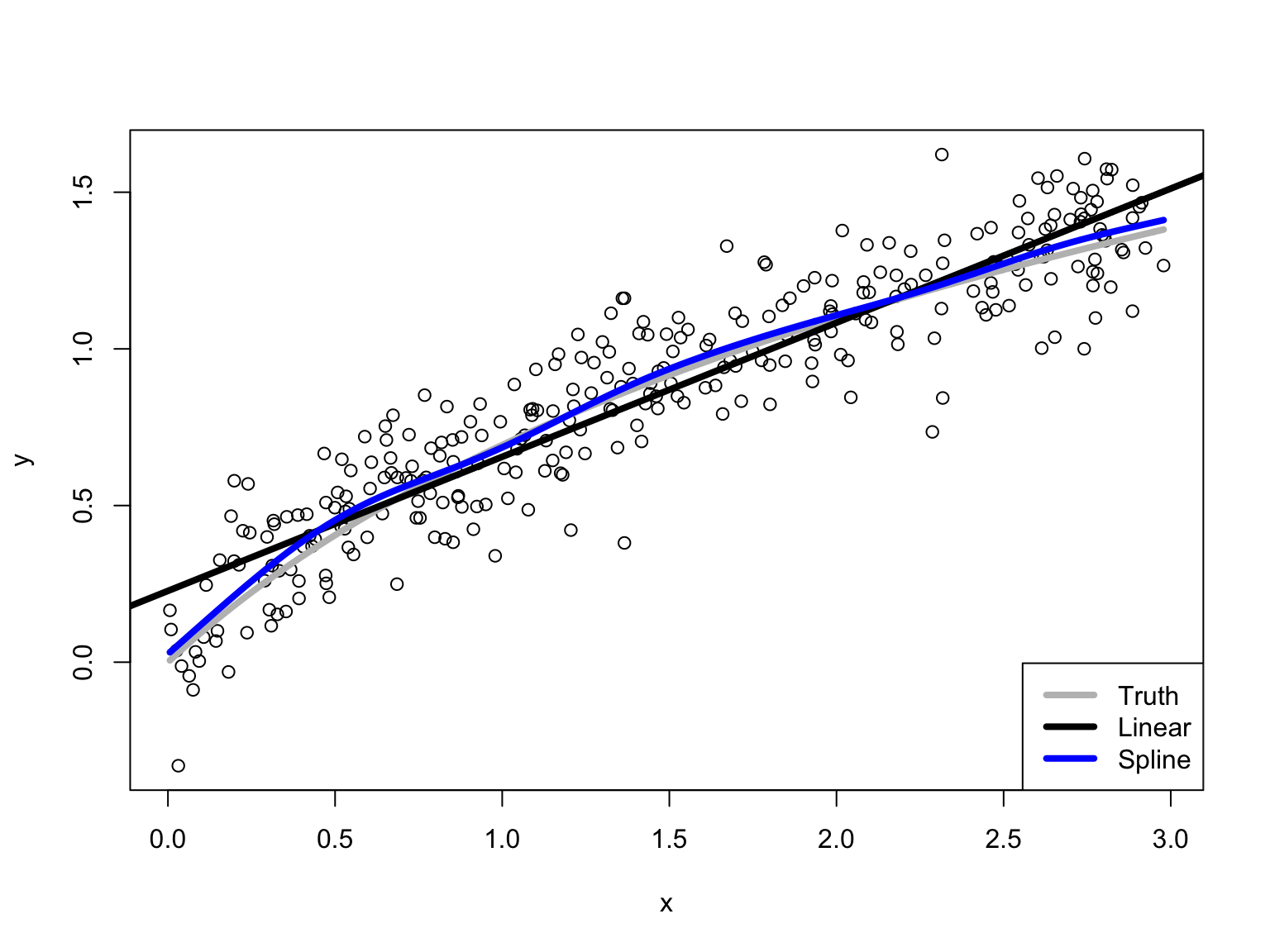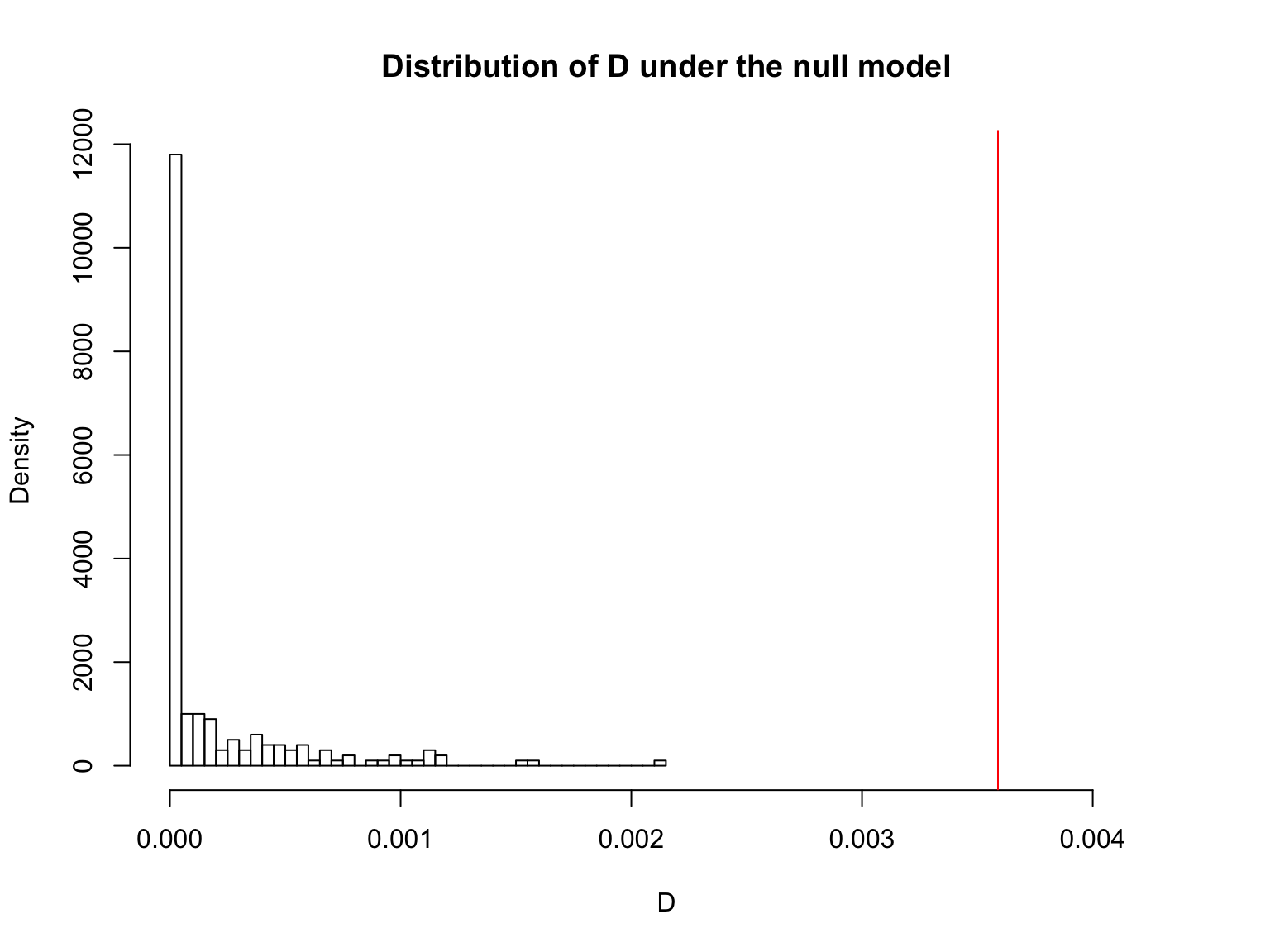# Testing a parametric regression model

21 February 2019

$\newcommand{\Expect}{\mathbb{E}\left[ #1 \right]}$

# The general problem

• You have a parametric regression model for your data
• e.g., linear with such-and-such variables
• You are worried that it might be misspecified, that the true $$\mu(x)$$ isn’t in the model
• Now that we know nonparametric regression, we can test this

# First case: linear specification is wrong

# Make up sample data
x <- sort(runif(300, 0, 3)) # Sorting simplifies plotting later
# Impose true regression function $\log{(x+1)} +$ Gaussian noise:
yg <- log(x+1) + rnorm(length(x), 0, 0.15)
# Bind into a data frame:
gframe <- data.frame(x=x, y=yg)

# What this looks like

Plot it, plus the true regression curve

plot(y~x, data=gframe, xlab="x", ylab="y", pch=16, cex=0.5)
curve(log(1+x), col="grey", add=TRUE, lwd=4)# What linear fit do we get?

Fit the linear model

glinfit <- lm(y~x, data=gframe)
print(summary(glinfit), signif.stars=FALSE, digits=2)
##
## Call:
## lm(formula = y ~ x, data = gframe)
##
## Residuals:
##    Min     1Q Median     3Q    Max
## -0.571 -0.098  0.011  0.105  0.401
##
## Coefficients:
##             Estimate Std. Error t value Pr(>|t|)
## (Intercept)    0.228      0.018      13   <2e-16
## x              0.428      0.011      40   <2e-16
##
## Residual standard error: 0.16 on 298 degrees of freedom
## Multiple R-squared:  0.84,   Adjusted R-squared:  0.84
## F-statistic: 1.6e+03 on 1 and 298 DF,  p-value: <2e-16

(N.B.: everything is crazily significant, but the linear model is wrong.)

# What the mis-specified linear fit looks like

plot(x, yg, xlab="x", ylab="y")
curve(log(1+x), col="grey", add=TRUE, lwd=4)
abline(glinfit, lwd=4)
legend("bottomright", legend=c("Truth", "Linear"),
col=c("grey", "black"), lwd=4)MSE of linear model, in-sample: 0.0251.

We’ll need to do that a lot, so make it a function:

(mse.residuals <- function(model) { mean(residuals(model)^2) })
## function(model) { mean(residuals(model)^2) }
• OFFLINE: Write comments giving the inputs and outputs of the function ms.residuals
• OFFLINE: Check that the function works (how?)

Fit the non-parametric alternative; use splines as implemented in gam():

library(mgcv)
gnpr <- gam(y~s(x),data=gframe)

Add the fitted values from the spline to the plot:

plot(x,yg,xlab="x",ylab="y")
abline(glinfit, lwd=4)
lines(x,fitted(gnpr),col="blue",lwd=4)
legend("bottomright", legend=c("Truth", "Linear", "Spline"),
col=c("grey", "black", "blue"), lwd=4)Calculate the difference in MSEs:

(D.hat <- mse.residuals(glinfit) - mse.residuals(gnpr))
##  0.003589289
• OFFLINE: Why do we believe this code works?

# How do we test the linear model?

• $$H_0$$: $$\mu(x)$$ is a linear function vs. $$H_A$$: $$\mu(x)$$ is not linear
• Test statistic: Difference in MSEs $$=D$$
• If the linear model is right, it will predict at least as well as a non-parametric model
• If the linear model is wrong, eventually the non-parametric model will beat it
• Need the sampling distribution of $$D$$ under the null hypothesis
• Need to simulate the linear model
• And need to calculate $$D$$ over and over

# Simulate the parametric model

Simulate from the parametric model, assuming Gaussian noise

sim.lm <- function(linfit, test.x) {
n <- length(test.x)
sim.frame <- data.frame(x=test.x)
sigma <- sqrt(mse.residuals(linfit))  # There are other ways to get sigma
y.sim <- predict(linfit,newdata=sim.frame)
y.sim <- y.sim + rnorm(n,0,sigma)
sim.frame <- data.frame(sim.frame,y=y.sim)
return(sim.frame)
}
• OFFLINE: Write comments describing input and output of the sim.lm function
• OFFLINE: How can we check that sim.lm is working?
• OFFLINE: How can we switch to non-Gaussian noise?

# Calculate the test statistic

Calculate difference in MSEs between parametric and nonparametric models on a data frame:

calc.D <- function(df) {
MSE.p <- mse.residuals(lm(y~x,data=df))
MSE.np <- mse.residuals(gam(y~s(x),data=df))
return(MSE.p - MSE.np)
}
• OFFLINE: Comments once again (with feeling this time)
• OFFLINE: Check that this function works properly (how?)

# Put the pieces together

Calculate the MSE difference on one simulation run:

calc.D(sim.lm(glinfit,x))
##  0.0001258959

Calculate the MSE difference on 200 simulation runs, so we get a sample from the null hypothesis:

null.samples.D <- replicate(200,calc.D(sim.lm(glinfit,x)))

How often does the simulation produce gaps bigger than what we really saw?

mean(null.samples.D >= D.hat)
##  0

# Look at the distribution

Plot histogram of the sampling distribution, and the observed value:

hist(null.samples.D,n=31,xlim=c(min(null.samples.D),1.2*D.hat),probability=TRUE,
main="Distribution of D under the null model",
xlab="D")
abline(v=D.hat, col="red")# Second case: linear model is properly specified

Deliberately left uncommented so that you can explore

y2 <- 0.2+0.5*x + rnorm(length(x),0,0.15)
# Why don't I have to make up x values again?
y2.frame <- data.frame(x=x,y=y2)
plot(x,y2,xlab="x",ylab="y",pch=16)
abline(0.2,0.5,col="grey",lwd=4)